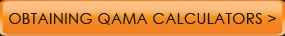﻿ The Scope of QAMAABOUT AIM INSTRUCTIONSESTIMATION GUIDE NEWS FEEDBACK CONTACTThe Scope of QAMA - Content The purpose of QAMA is to instill and verify Quantitative comprehension Understanding the meaning of fundamental functions (as encountered in high school) by themselves It is not possible, and thus, not intended, to include the gamut of mathematical relations. It is therefore not viable to include only some cases e.g. zero tolerance for, say, sin2x+cos2x,  tanx - sinx/cosx,  (400- 81)/29  (which must be 11 – from (a+b)(a-b)=a2-b2 ) etc. because critics would then suspect QAMA of not knowing about the rest… Complex numbers and statistical functions are not included because it is assumed that if students had been using QAMA until that stage, they would already have developed the correct attitude of showing interest in meanings and values (just as one would not introduce the concepts of physical fitness to people showing up for a Marathon).    Rational and mixed Fractions: The question about their inclusion arises only regarding whether the  estimate  can be entered as rational fraction. (In the task a rational fraction can be entered as n/m, and a mixed fraction as (n +m/k) - which also helps to remind what this is about.)  They are excluded as estimates because: - There is little scope for approximate rational fractions: It is easier to appraise/visualize a fraction as a decimal (with the exception, perhaps, of 1/2, 1/3  & 1/4) So, the only scope for entering an estimate as a rational fractions is  if  one knew the exact result. This, however, results usually from the purely mechanical performance of the ‘common denominator gymnastics’ which few students ever understand why it works, and more seriously: the fact that “5/3 + 7/5 equals 46/15” is rarely bound to any ‘quantitative insight’. Still: If one does wish to express the result as a rational fraction i.e. n/m, one can multiply the task by m and enter n as the estimate of the result. This would also prove some insight into the use of rational fractions)
 ```©2012 QAMA LLC. ALL RIGHTS RESERVED US Patent 6820800```